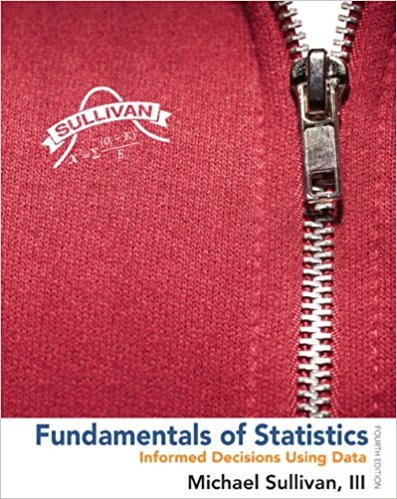×
×

# Solved: The Empirical Rule The following data representISBN: 9780321838704 30

## Solution for problem 23AYU Chapter Chapter 3.2

Fundamentals of Statistics | 4th Edition

• Textbook Solutions
• 2901 Step-by-step solutions solved by professors and subject experts
• Get 24/7 help from StudySoup virtual teaching assistantsFundamentals of Statistics | 4th Edition

4 5 1 278 Reviews
12
5
Problem 23AYU

The Empirical Rule The following data represent the weights (in grams) of a random sample of 50 M&M plain candies.

 0.87 0.88 0.82 0.9 0.9 0.84 0.84 0.91 0.94 0.86 0.86 0.86 0.88 0.87 0.89 0.91 0.86 0.87 0.93 0.88 0.83 0.95 0.87 0.93 0.91 0.85 0.91 0.91 0.86 0.89 0.87 0.84 0.88 0.88 0.89 0.79 0.82 0.83 0.9 0.88 0.84 0.93 0.81 0.9 0.88 0.92 0.85 0.84 0.84 0.86

(a) Determine the sample standard deviation weight. Express your answer rounded to three decimal places.

(b) On the basis of the histogram drawn in Section, Problem, comment on the appropriateness of using the Empirical Rule to make any general statements about the weights of M&Ms.

(c) Use the Empirical Rule to determine the percentage of M&Ms with weights between 0.803 and 0.947 gram. Hint:= 0.875.

(d) Determine the actual percentage of M&Ms that weigh between 0.803 and 0.947 gram, inclusive.

(e) Use the Empirical Rule to determine the percentage of M&Ms with weights more than 0.911 gram.

(f ) Determine the actual percentage of M&Ms that weigh more than 0.911 gram.

Problem

Rates of Return of Stocks Stocks may be categorized by industry. The data on the following page represent the 5-year rates of return (in percent) for a sample of consumer goods stocks and energy stocks ending November 10, 2010.

(a) Determine the mean and the median rate of return for each industry. Which sector has the higher mean rate of return? Which sector has the higher median rate of return?

(b) Determine the standard deviation for each industry. In finance, the standard deviation rate of return is called risk. Typically, an investor “pays” for a higher return by accepting more risk. Is the investor paying for higher returns in this instance? Do you think the higher returns are worth the cost?

 CONSUMER GOODS STOCKS 9.83 10.11 11.06 9.32 7.5 8.66 13.7 15.04 17.24 16.83 6.22 8.86 11.79 9.64 -14.12 16.83 14.85 15.24 34.17 -8.32 0.52
Step-by-Step Solution:

Step 1</p>

a)

First we need to find mean.=== 0.766

Here standard deviation is= 0.0012

Then the sample variance is

s=s =s = 0.036

Hence the sample variance is s = 0.036 gram.

b)

The histogram is given below.The histogram is approximately symmetric, so the Empirical Rule is applicable.

c)

95% of the M&Ms should weigh between 0.803 and 0.947 gram.

d)

96% of the M&Ms actually weigh between 0.803 and 0.947 gram.

e)

16% of the M&Ms should weigh more than 0.911 gram.

f)

12% of the M&Ms actually weigh more than 0.911 gram.

Problem

Step 2 of 2

##### ISBN: 9780321838704

Unlock Textbook Solution